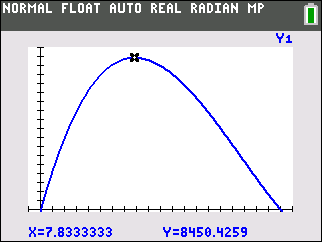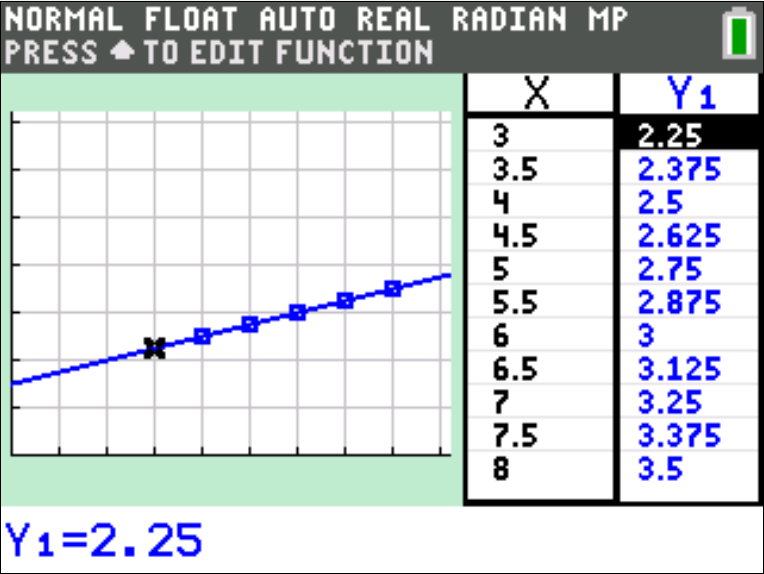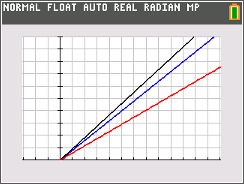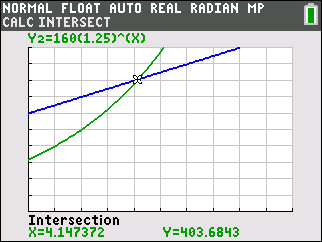Creating Boxes

Students graph the relationship between the length of the sides of the cut-out squares and the volume of the resulting box. They trace the graph to decide the best square-size which can result in a box of maximum volume.
•TI-84 Plus CE
•TI-84 Plus C Silver Edition
• TI-84 Plus Silver Edition
• TI-84 PlusThe Variables of Renting

In this activity, students will identify what a variable is, construct a table of values, graph the ordered pairs from the table, and use the TI-84 Plus C Silver Edition to graph the equation.

•TI-84 Plus CE
•TI-84 Plus C Silver Edition
• TI-84 Plus Silver Edition
• TI-84 PlusShark Frenzy

Students examine equations in the family of linear functions which are of the form y = m x, each of which correspond to a different family of sharks. They relate the slope to the ratio of the shark’s fork length to its total length. When comparing two sharks of the same length, students conclude that slopes of the lines of the three families decrease with increasing length of the upper caudal lobe
•TI-84 Plus CE
•TI-84 Plus C Silver Edition
• TI-84 Plus Silver Edition
• TI-84 PlusGuppies and Frogs

In this activity, students will create equations with two or more variables to represent relationships between quantities. They will also graph equations on coordinate axes with labels and scales.
•TI-84 Plus CE
•TI-84 Plus C Silver Edition
• TI-84 Plus Silver Edition
• TI-84 Plus••